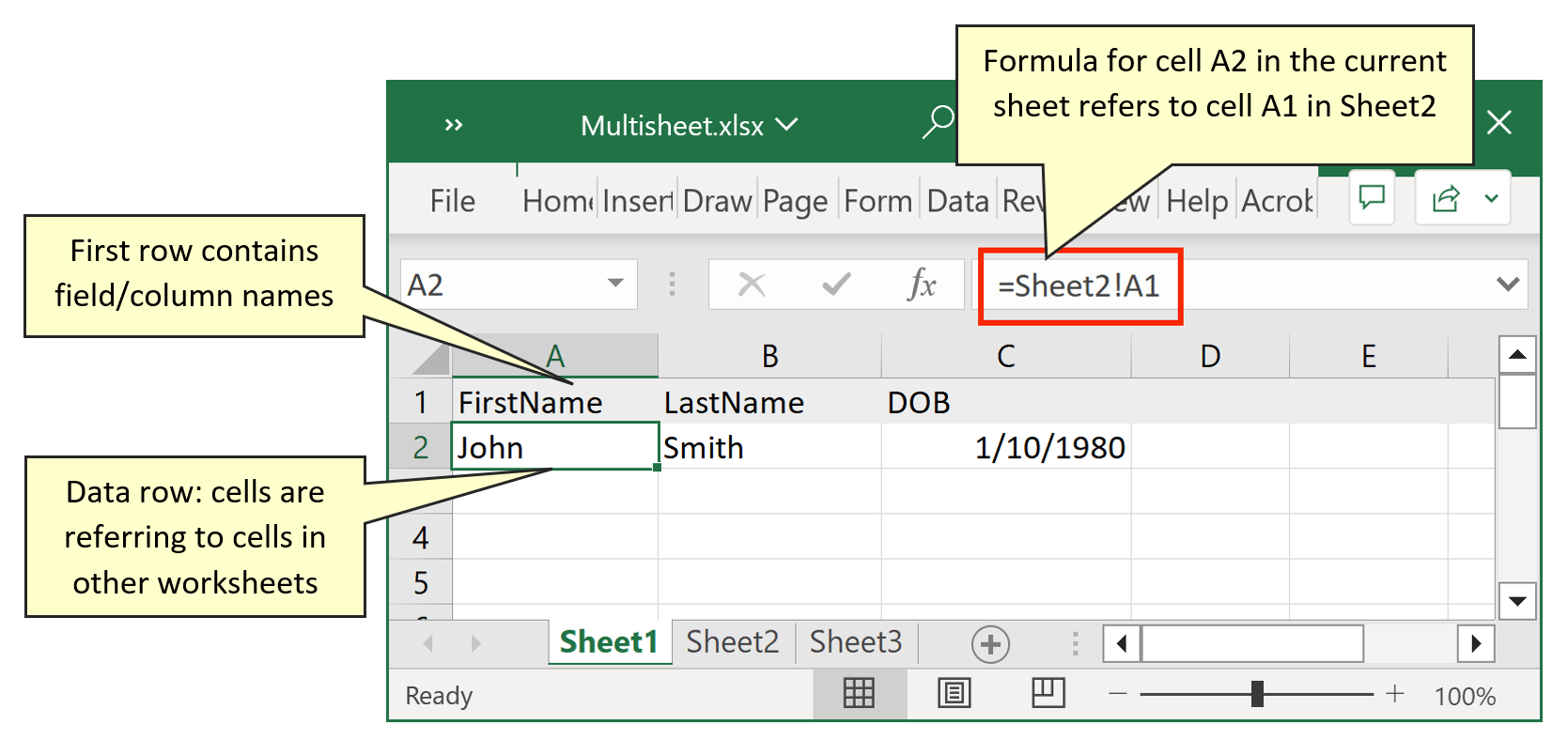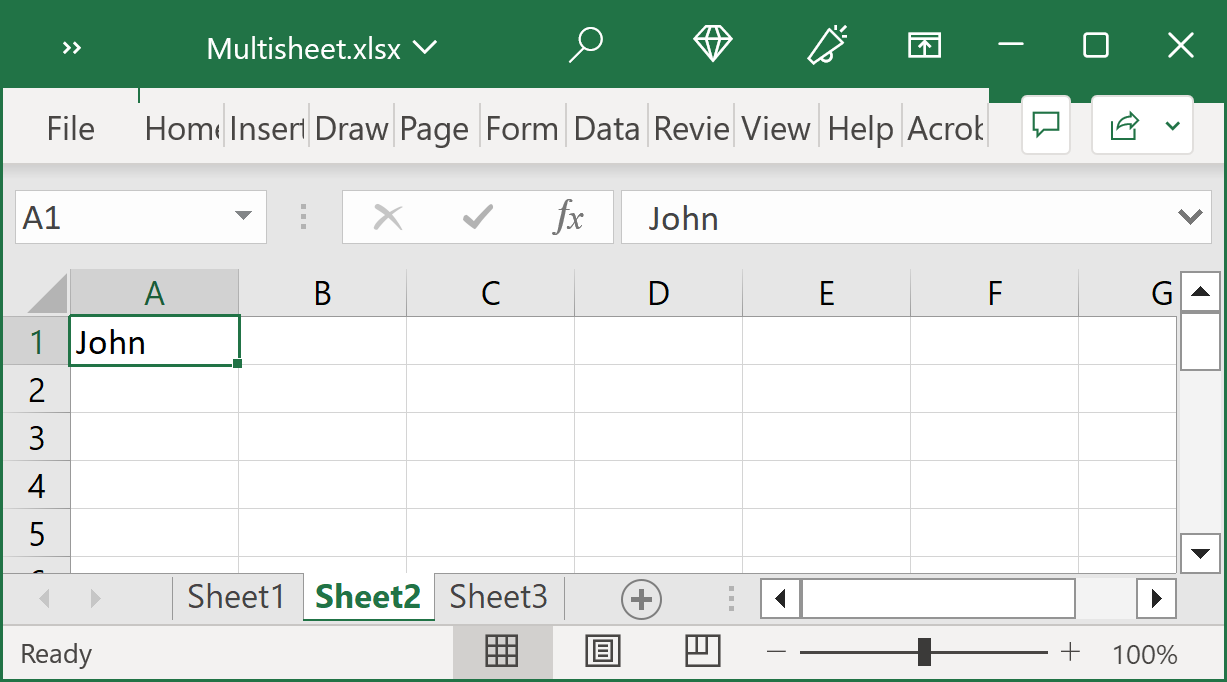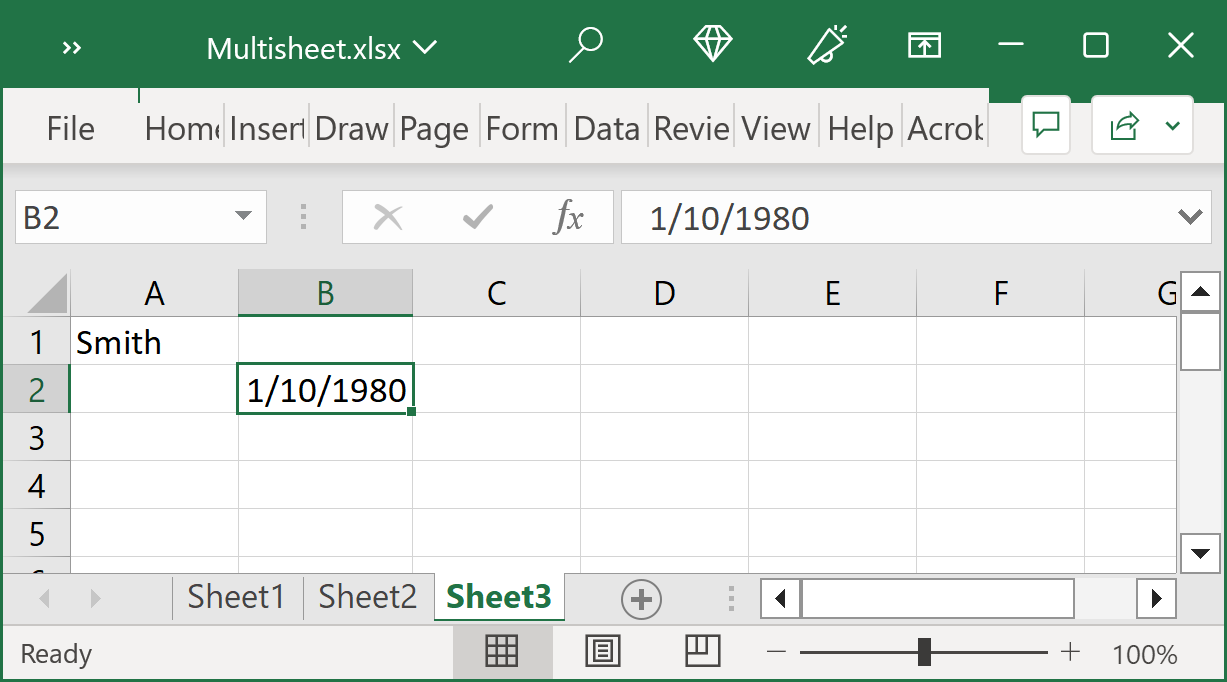# Using Data from Multiple Excel Worksheets to Populate a PDF Form

## Tutorial for AutoMailMerge plug-in for Adobe® Acrobat®

Introduction
Sometimes, it is necessary to fill out a single copy of PDF form by taking data from cells located in several worksheets of the same Excel workbook. The data is not necessarily organized in a tabular form as required by the mail merge process. It is still possible to use AutoMailMerge plug-in to accomplish this task with a little modification to the data file. The approach is to create a new worksheet that is organized in a tabular form and add a row of cells that refer to values located in another worksheets.
Referring to a Cell in Another Worksheet
In Excel, use formulas to refer to data values located on another worksheets. You can refer to cells that are on other worksheets in the same workbook by prepending the name of the worksheet followed by an exclamation point (!) to the start of the cell reference. For example, use =Sheet2!A1 to refer to cell A1 located on Sheet2. This formula will return a value of cell A1 located on worksheet Sheet2. If you change the value of cell A1 on Sheet2, it will be also get automatically updated in the cell that uses this formula.
The same method can be used with functions and page ranges. In the following example, the worksheet function named SUM calculates the sum of all values for the range B1:B10 on the worksheet named Accounting in the same workbook: =Accounting!B1:B10.
Sample Use Case
In the following example, Sheet1 worksheet is used to present data from two other worksheets Sheet2 and Sheet3 in a tabular form. The first row of the worksheet contain field names for corresponding columns, while a second row contains data cells. Each cell on a second row uses a formula to get a value from another worksheet.Here is a list of formulas used for the second row cells:
• FirstName column: =Sheet2!A1
• LastName column: =Sheet3!A1
• DOB column: =Sheet3!B2
Here are the screenshots of Sheet2 and Sheet3 worksheets where actual data is located:Running a Mail Merge
Now it is possible to run a regular mail merge job using AutoMailMerge plug-in while selecting Sheet1 as input data source for the mail merge. Each data row in Sheet1 worksheet will be used to populate a single copy of the input PDF form.